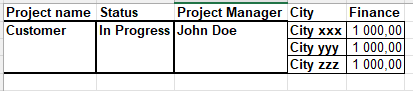# Report where I can present the finance of one project (issue)

"Hi, I’m trying to create a report where I can present the finance of one project (issue).
The problem is that the Finance value (1000) duplicates itself (it should be 1000 for all the cities selected in total, not 1000 for each and one of them).Can you please help me with suggestion how we could solve this? The City row is from a multi-selection field that we use.

Thank you."

Hi @Coachen ,
In the case of multi-select fields, this is expected behavior when the measure is used.
One of the options is to use the issue property “Issue city” to see all values in one cell. In my example I am usign “Issue labels” property:

Or you can divide the value “Finance” from the issues with the member count of your “City” dimension. You can create a custom measure in Measure dimension using this formula to count how many members are per issue (using “Labels” as example, but you need to use your “City” dimension):

``````NonZero(
Count(
Filter(
Descendants(
[Label].[Label].Members),
[Label].CurrentHierarchyMember.Name<>"(none)"
AND
[Measures].[Finance]>0
)
))
``````

An then use that count to divide the measue:

``````IIf(
[Measures].[Count labels]>0,
CoalesceEmpty([Measures].[Finance],0)/
[Measures].[Count labels],
[Measures].[Finance]
)
``````

In the report:

Best,
Gerda // support@eazyBI.com